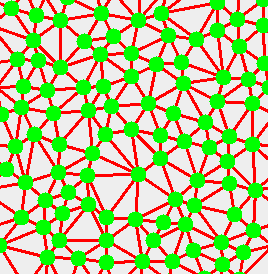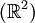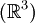# Delaunay simplexesA Delaunay simplex is the dual of the Voronoi diagram. Delaunay simplexes were developed by Борис Николаевич Делоне. In two-dimensions$({\mathbb R}^2)$ it is more commonly known as Delaunay triangulation, and in three-dimensions$({\mathbb R}^3)$, as Delaunay tetrahedralisation.